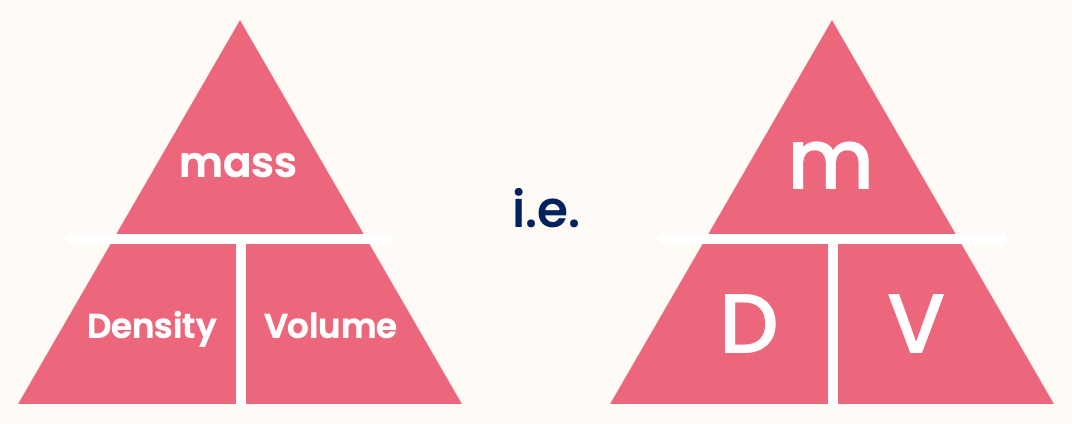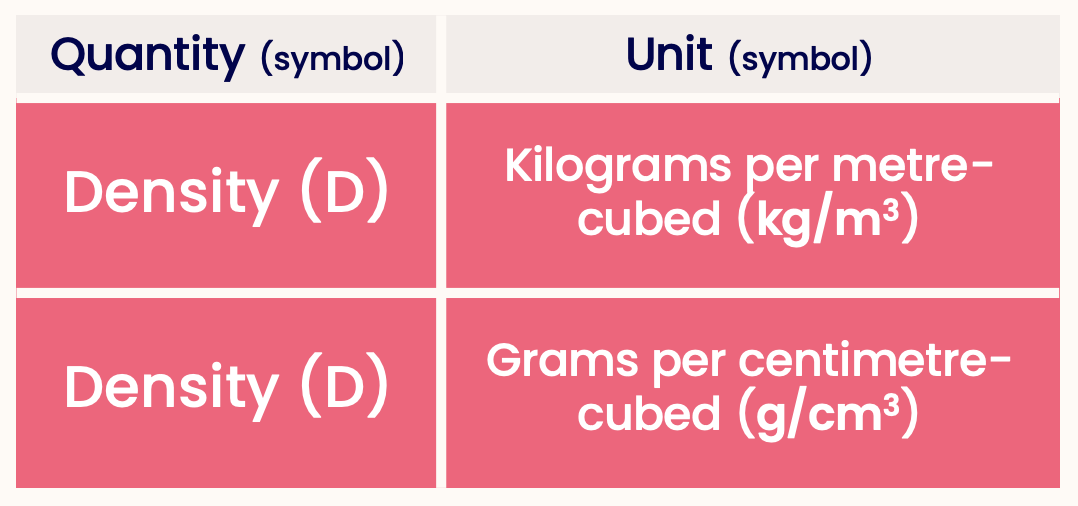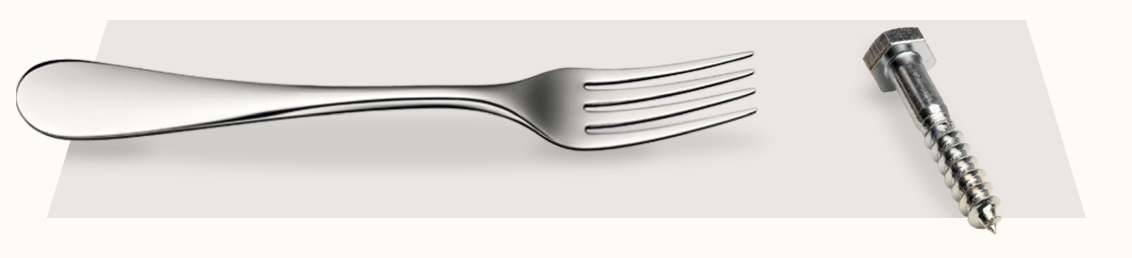Physics
Fox

### Summary

• The density of an object is defined as its mass divided by its volume. $\text{Density} = \frac{ \text{Mass} }{ \text{Volume} }$

• This can be written on a formula triangle as:• The units of density are kg/m3 ("kilograms per metre-cubed") and g/cm3 ("grams per centimetre-cubed"). When answering questions, use whichever one is easiest!• All solids and liquids made from the same material will have the same density.This iron fork and iron screw both have same density.

• If an object is less dense than the liquid/gas it is in, it will float.Wood floats in water, a helium balloon floats in air.

• If an object is more dense than the liquid/gas it is in, it will sink.Gold coins sink in water, an elephant (or basically anything) sinks in air!

Quiz

### Congratulations!

14 of 14 questions completed

+ ⭐️ collected.

• You can change your display name at any time.
• at least 1 letter
• at least 1 number
• at least 8 characters